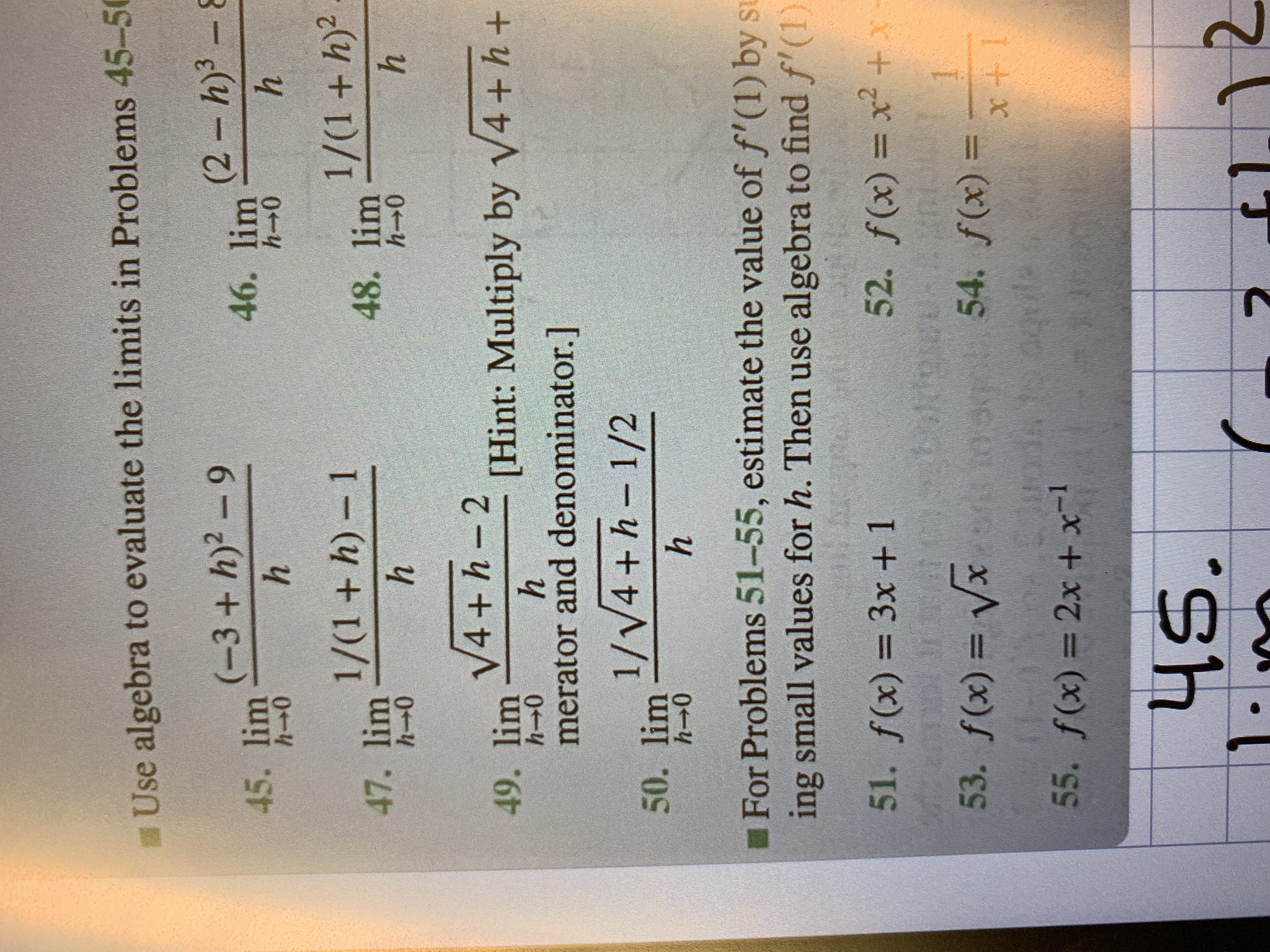# Use algebra to evaluate the limits in Problems 45-5045. lim3+ h)2 946. lim 2-h)3 - 8hhh- 0h- 048. lim(1+h)2h-047. lim1+h) -hh- 0h49. lim V4h-2h- 0[Hint: Multiply by V4 + h+hmerator and denominator.]50. lim V4 + h- 1/2h 0For Problems 51-55, estimate the value of f'(1) by suing small values for h. Then use algebra to find f (1)51. f(x) 3x +152. f(x) x2+x1o:54. f(x)=53. f(x)=55. f(x) 2x+x145.12

Question
13 views

50.help_outlineImage TranscriptioncloseUse algebra to evaluate the limits in Problems 45-50 45. lim3+ h)2 9 46. lim 2-h)3 - 8 h h h- 0 h- 0 48. lim(1+h)2 h-0 47. lim1+h) - h h- 0 h 49. lim V4h-2 h- 0 [Hint: Multiply by V4 + h+ h merator and denominator.] 50. lim V4 + h- 1/2 h 0 For Problems 51-55, estimate the value of f'(1) by su ing small values for h. Then use algebra to find f (1) 51. f(x) 3x +1 52. f(x) x2+x 1 o:54. f(x)= 53. f(x)= 55. f(x) 2x+x1 45. 12 fullscreen
check_circle

Step 1

The given lim...

### Want to see the full answer?

See Solution

#### Want to see this answer and more?

Solutions are written by subject experts who are available 24/7. Questions are typically answered within 1 hour.*

See Solution
*Response times may vary by subject and question.
Tagged in

### Limits